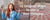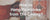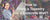0

# Learn how to Read the Time on a Clock

In today's world, we are obliged to know how to read the time, it is an indispensable and precious skill that we must master because our lives are punctuated by time. We measure time in many ways. On a clock, time is indicated in hours and minutes (and sometimes even seconds). On a calendar, we determine time in days, weeks, months and years. In this article, we will learn how to read the time using the minutes and hours of a classic clock.

## 1- Understanding the dial of a clock

A clock is a man-made device to indicate the time of day. On the clock are 12 numbers. Each of these numbers will be useful to set the hours and minutes.

For the small hand: the numbers indicate the hours and it advances by one number every hour.
For the large hand: the numbers indicate the minutes. It moves one notch every minute and takes 5 minutes to reach the next number. Each number represents 5 minutes. The full turn of the big hand means that one hour has passed.
The dial of an analog clock looks like the pictures below:

The numbers in blue represent the numbers on all analog clocks.
The numbers appearing in orange (outside the dial) are not present on conventional clocks. However, they will be used to determine the minutes more precisely. For a question of space and aesthetics, all these numbers cannot appear on the dial. To overcome this problem, only a few numbers appear on the clock. You will see on a clock only the multiples of 5 (i.e.: 5, 10, 15, 20...up to 55).

Now we will add the hour and minute hands to this dial. Don't forget that the hour hand is the shortest and the minute hand the longest. We are going to set our clock to indicate 1 hour.

## 2- Reading the hours on a clock

Reading a clock is very simple, you just have to understand how it works.

To start, you have to locate the hour hand, it goes from one number to another every hour and makes a complete turn of the dial every 12 hours, so twice a day (because on a classic clock there are only 12 numbers).

Look at the number which is pointed by the arrow of the small hand, it will give you the time. Often the arrow is not exactly in front of the number. In this case, you have to take the last number passed by this hand.

For example, if the hour hand is halfway between 3 and 4 then you will keep the number 3 for the hours. Always in this case, even if the arrow is closer to the number 4, you will always take the number 3 as long as the 4 has not been reached or passed.

Here is a new example illustrated below : Here you can see that the hour hand is a little more than halfway between 1 and 2. Even if it is closer to 2, we will still say that 1 is the hour number because the hour hand has not reached 2.

## 3- Reading the minutes on a clock

Now let's talk about the minute hand. We remind you that the minute hand is the longer of the two hands.

It indicates the minutes that have elapsed since the start of a new hour.
The minute hand rotates fully every hour.

If the minute hand points to a number on the dial, simply multiply it by 5 and you will get the number of minutes that have passed since the beginning of the hour.

For example, if the minute hand points to the number 1, it corresponds to 5 minutes (the calculation is 1x5).

Here is a second example, if the minute hand points to number 6 (the calculation is 6x5) it corresponds to 30 minutes.

 1 2 3 4 5 6 7 8 9 10 11 12 5min 10min 15min 20min 25min 30min 35min 40min 45min 50min 55min 0min

When the minute hand is between two numbers then take the last number (blue) passed by the big arrow and add the number of graduation from this number (blue) to the position of the hand.

Let's take the example above: The minute hand is between 7 and 8. We know that the number 7 is equal to 35 minutes (see table above). Next, you estimate where the arrow is actually pointing. Clocks have small slashes (graduations) to help you count. In our example, we have the orange numbers written on the edge to make the minutes easier to read.

The minute hand points to 38 (on a regular clock you will notice that this is the third mark after 7, so just add 3 to 35 and you get 38. Here since the orange numbers are present, we can clearly see that the number 38 is pointed.

So the exact time is 1:38, which reads "one hour, thirty-eight minutes".

Now that you know how to read the time on a clock, come and discover our wall clock store. On the site, you will find a very large choice of clocks which in addition to giving you the time will bring a decorative touch to your interior! There is something for all tastes!

Comments will be approved before showing up.

### Also in My wall Clock Blog##### 12 Original Ways to use a Wall Hanging

The following twelve ways to use your hanging will help you get the most out of it. This list is not exhaustive, so you can use your imagination to find other original ways to use your new hangings and give a second life to the old ones!##### How to Hang Tapestries from The Ceiling?